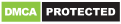# HiEdu Scientific Calculator : He-570

Genres
Version
4.3.1
Developer
-
Requires
Android 4.1+
Updated
2021-04-22HiEdu Scientific Calculator: He-570: A powerful calculator app that includes calculator for math, calculator for money, calculator equation… This is a calculator app which has a lot of features such as: scientific calculator, standard calculator, complex, programmer, table, matrix, vector, graph, solve the equation, converter, math formulas, physical formulas, chemistry. It is a very useful tool for education to help complete complex math, algebra, or geometry problems.

KEY FEATURES
Scientific calculator
• It is a scientific calculator app supports most of the features of real calculators: calculator for fractions, calculator algebra, calculator derivative, calculate integral, calculate differential, calculator square root, calculator with exponents, calculator with log, calculator with remainder, calculator division, calculator with variables,.. In addition, the calculator has factorial, absolute, combination, permutation… help you calculate easily.
• Natural Display makes it possible to input and display fractions and certain functions (log, x2, x3, x^), “, x−1, 10^, e^ , d/dx, Σ, Abs …) just as they are written in your textbook.
• Save history, select a calculation in list history and edit it.
• Create favorite calculations that make calculations faster.

Standard calculator
• This is a perfect tool for daily calculations such as: independent memory (M+, M-), figuring percentages with memory functions similar to a small handheld calculator.
• Income Tax Calculator : income tax personal, income tax calculation, sales tax calculator, tax return calculator.
• Save history, Select a calculation in list history and edit it.

Complex number calculation
Complex is useful to find a complex number as a result of addition, real part of complex number, imaginary part of complex number, subtraction complex numbers calculator, multiplying complex numbers calculator and dividing complex numbers calculator.

Matrix calculator
It’s main task – calculate mathematical matrices.
Supported matrix operations: matrix inverse calculator, matrix determinant, matrix addition, matrix subtraction, matrix multiplication, matrix transposition.

Vector calculation
This calculator app has many functions: vector calculation, calculate cross product, calculate dot product, unit vector, find angle between two vectors.

Graphing calculator
• Easily graph functions such as: drawing graph, graphing calculators, find special points of functions.

Solve the equation
• Solve cubic equations
• Solve inequalities
• Solve the system of equations

Converter
• Available unit conversions include:
+ Currency conversion
+ Temperature converter
+ Length converter
+ Mass/Weight
+ Speed converter
+ Area conversion
+ Cooking Volume
+ Pressure converter
+ Power converter
+ Energy
+ Time converter
+ Fuel Consumption converter
+ Digital Storage conversion

Mathematics formulas
• This app has 1000+ math formula and more to come.
• Now no need to make paper notes to remember mathematics formulas just have this app put all the formulas on your favourite phones.
• you’ll find formulas very simply explained in app with necessary figures will help you to understand very easily.

Physics formulas
• The application is a physical handbook. Contains most physical formulas for students and undergraduate help users quickly refer to any Physics formulas for their study and work.This app displays most popular as well as advanced formulas in seven categories:

Chemical reactions
• Allows to discover chemical reactions and to solve the chemical equations with one and several unknown variables.

Important Note : Our app products are not related to physical calculators such as casio scientific calculator, hp scientific calculators, texas scientific calculators… The app was developed by the research of the company team.
+ Unlock keyboard pro.
+ Added italian language. (Thanks to “Stefano Macrina”)

Recommended for you

You may also like